Home / Circuits / Mesh Analysis for AC Circuits

# Mesh Analysis for AC Circuits

Similar to Nodal Analysis, Mesh Analysis for both DC and AC circuits are similar. The only difference is that in AC, we are dealing with impedances instead of just resistors. The aim of this tutorial is to make Mesh Analysis for AC circuits simpler for you.

### Introduction to Mesh Analysis

Mesh analysis is a result of simplifying Kirchoff’s Voltage Law (KVL). Recall that according to KVL, the algebraic sum of voltages inside a loop is zero.

Consider the following loop: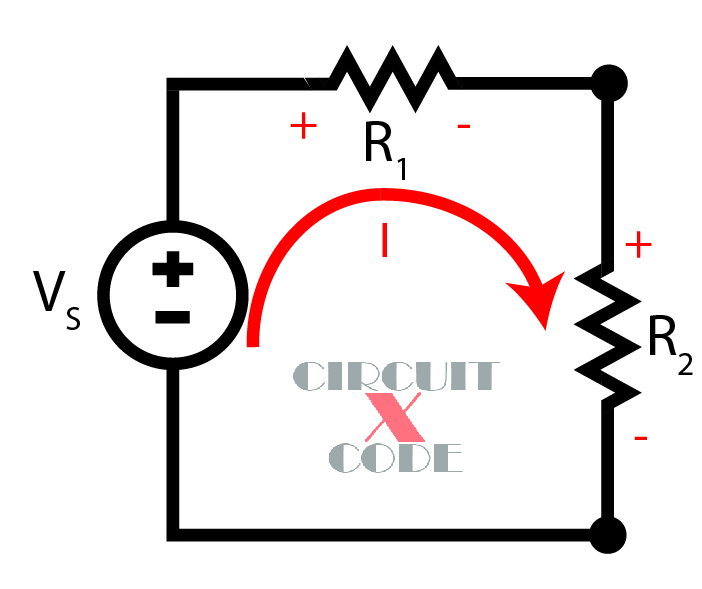In the loop and for any passive circuit, there are sources and sinks. Sources produce energy while sinks consume energy. In the circuit above, we have one voltage source and two resistors as sinks. We assign a convention where sources have positive signs while sinks have negative signs: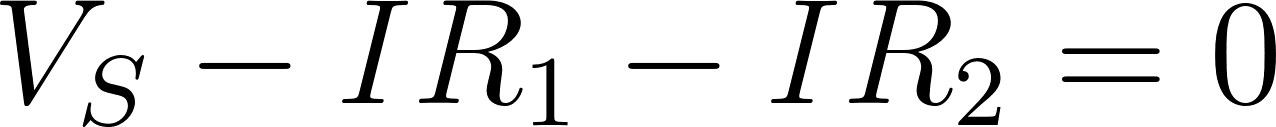The sign on the sources and sinks have significance: if the loop is traversed from minus to plus, the sign is positive, otherwise, the sign is negative.

Now, when the loop is adjacent to another loop, two currents now pass through an element: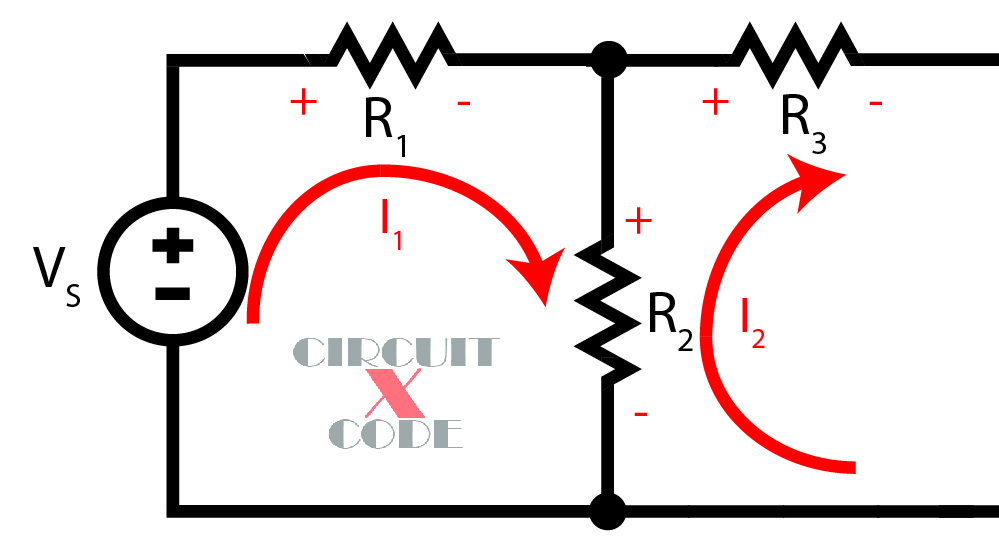The loop direction for all mesh currents is always assumed to be the same. Here, both I1 and I2 are in clockwise direction.

The voltage across the resistor R2 will be: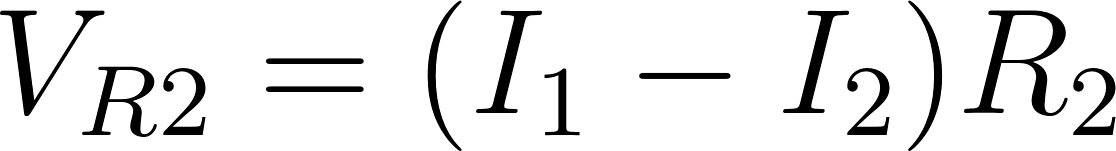The expression I1 – I2 here is derived by looking at the junction above R2. The currents in that junction would be: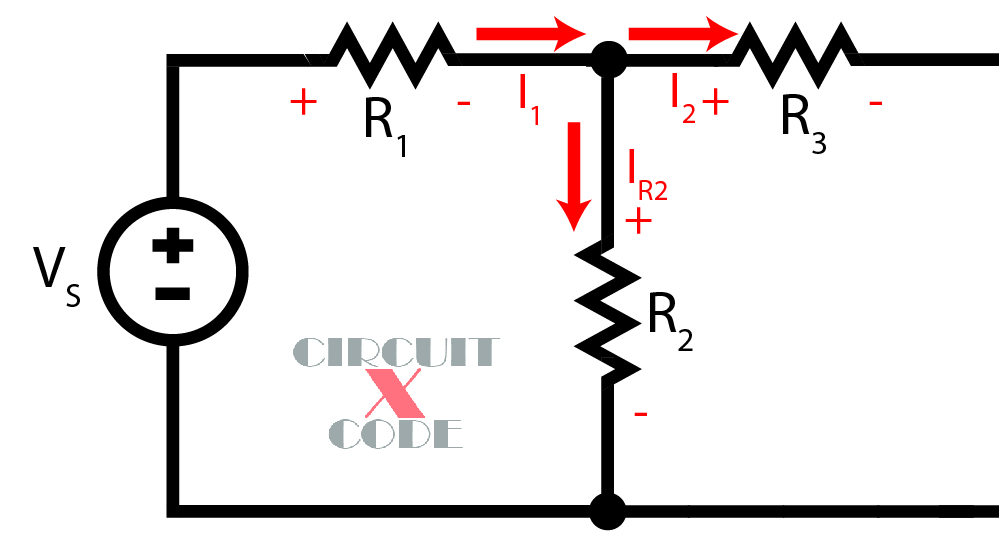By KCL, the current equation is: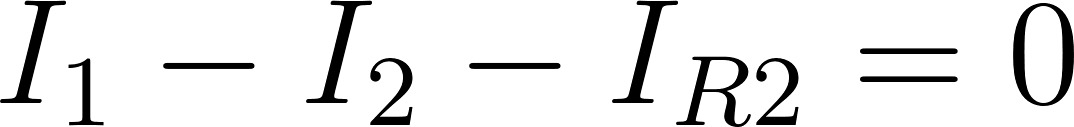Hence, the current across R2 is: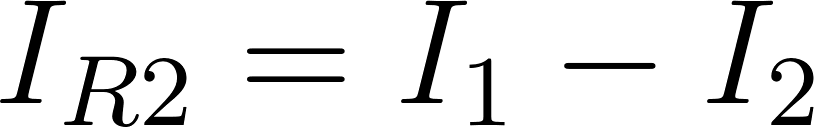Now if we do KVL for the two adjacent loops, our equation for the first loop will be:Similarly, the equation for the second loop (assuming R2 and R3 are the only elements)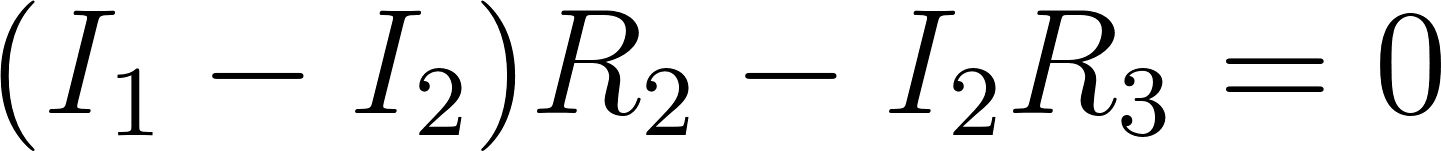I1-I2 here is now positive because the loop is traversed (looking at I2) from minus to plus across R2.

Normally, the values of the voltage source and resistances are given. Thus, we have two equations with two unknowns.The aim is to arrive at a number of mesh equations for a given number of unknown currents. Generally, the number of unknown mesh currents is equal to the number of loops in the circuit. We then use any system of linear equation techniques to solve for the unknowns.

### Mesh Analysis for AC

As mentioned, the only difference between mesh analysis in DC and AC circuits is that AC circuits deal with impedances (capacitive, inductive, or resistive). Consider the circuit below: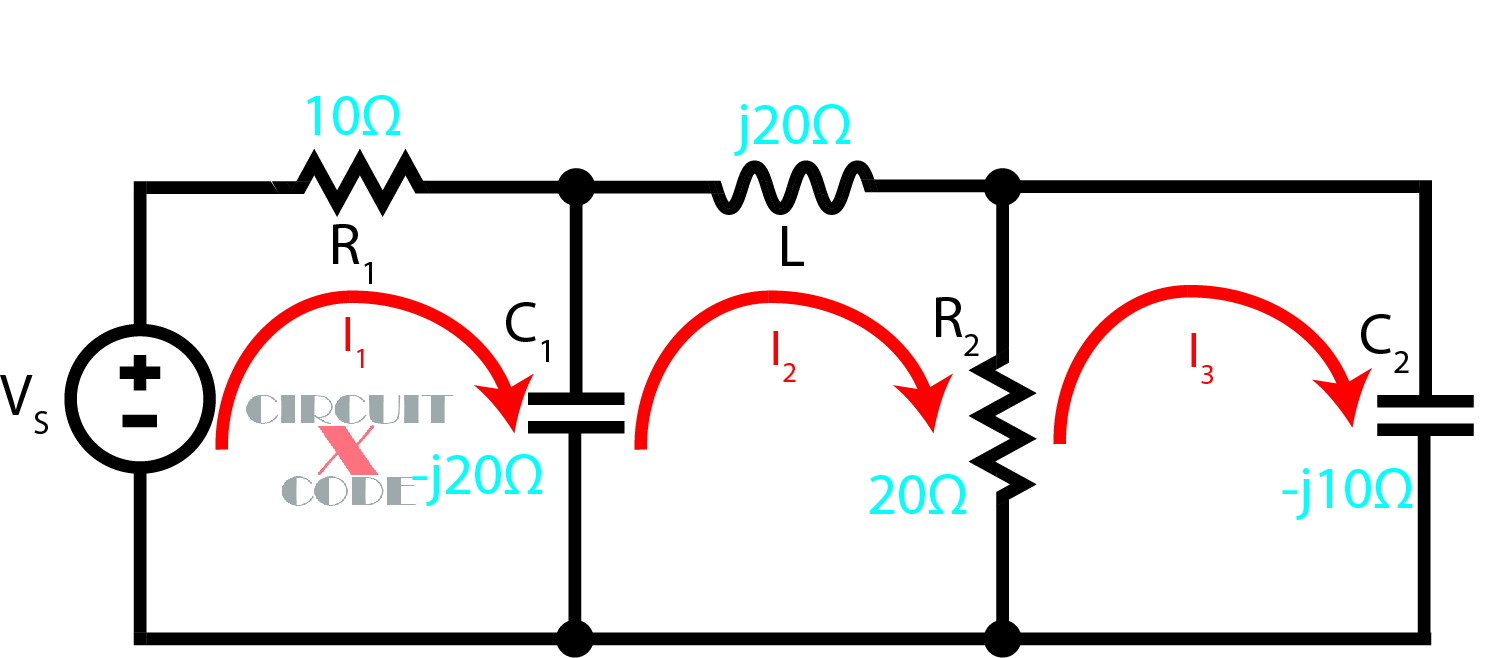This circuit has resistors, capacitors and inductors expressed as phasor elements. Let’s try to solve for the voltage across C1.

At the first loop, the equation is:Here’s the equation on the second loop:Finally, here’s the equation for the third loop: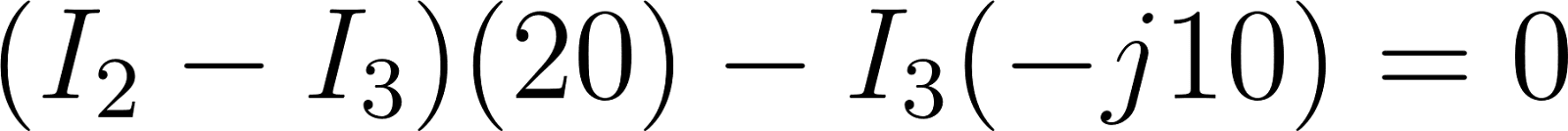Simplifying, our three equations are now: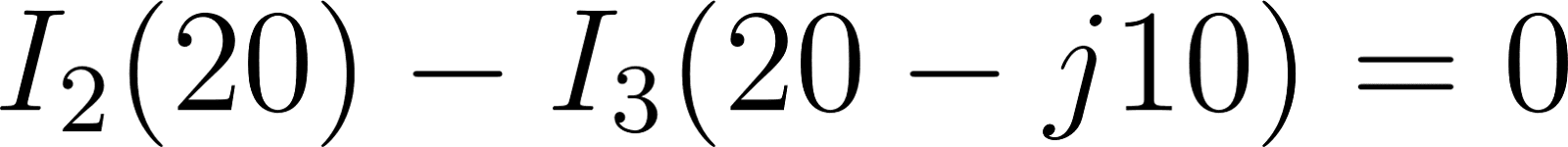We then use techniques like Cramer’s Rule to solve for the first and second loop current. Why these two currents? Because the voltage across the capacitor would be: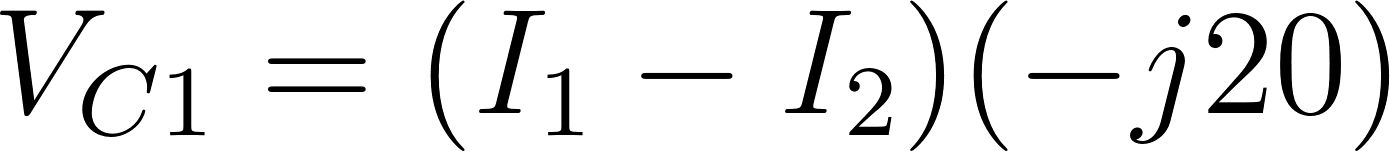### A Mnemonic for Mesh Analysis

We can use a general statement to easily recall mesh analysis for AC:

“Sum of impedances on the loop, multiplied by the loop current, minus the sum of impedances common to this loop and the next loop multiplied by the next loop current is equal to the sum of applied voltages to this loop”

Let’s see if this statement applies to our mesh equations. Let us look back to our AC circuit:The first part is:

“Sum of impedances on the loop, multiplied by the loop current…”

Assuming this is referring to the 1st loop then:The next statement,

“minus the sum of impedances common to this loop and the next loop multiplied by the next loop current…”

This should be: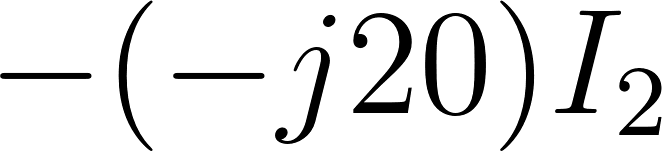Finally,

“…is equal to the sum of applied voltages to this loop”

The applied voltage here is any voltage source within the loop. There is just one voltage source in the first loop thus,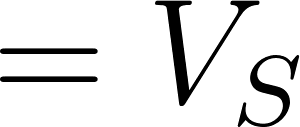Altogether, the equation for the first loop is:This is the same as the first mesh equation, just rearranged!

We can also apply the same statement to the second and third mesh:Again, this is the same equation we arrived on earlier.

### Conclusion

Hopefully, this tutorial achieved its objective which is to make you understand mesh analysis for AC circuits. For questions, reactions and suggestions, please drop a comment below!

## Analog to Digital Conversion Calculator

Analog to Digital Conversion Overview Microcontrollers are essentially computers. And like computers, they only understand …